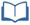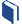# Predicting the Future

Estimated Time: 90 minutes

###Lesson Objectives:

• The students will collect simple random samples from surveys.
• The students will make predictions from a sample.

###Resources for this lesson:

Before you begin, download your Algebra II Journalfor Predicting the Future. You will be completing activities in the journal throughout this lesson.

Visit this section on each page to access information about key terms and other resources to help facilitate the lesson.

> Glossary> Calculator Resources> Teacher Resources: Instructional NotesKey Common Core State Standards:
S.IC.1: Understand statistics as a process for making inferences about population parameters based on a random sample from that population.

Supporting Common Core State Standards:
7.SP.1: Understand that statistics can be used to gain information about a population by examining a sample of the population; generalizations about a population from a sample are valid only if the sample is representative of that population. Understand that random sampling tends to produce representative samples and support valid inferences.

7.SP.2: Use data from a random sample to draw inferences about a population with an unknown characteristic of interest. Generate multiple samples (or simulated samples) of the same size to gauge the variation in estimates or predictions. For example, estimate the mean word length in a book by randomly sampling words from the book; predict the winner of a school election based on randomly sampled survey data. Gauge how far off the estimate or prediction might be.

Standards for Mathematical Practice Emphasized:

1. Make sense of problems and persevere in solving them.
2. Reason abstractly and quantitatively.
3. Construct viable arguments and critique the reasoning of others.
4. Model with mathematics.
5. Use appropriate tools strategically.
8. Look for and express regularity in repeated reasoning.

Next >